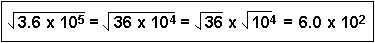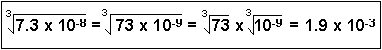Math Skills ReviewScientific Notation

Scientific notation is the way that scientists easily handle very large numbers or very small numbers. For example, instead of writing 0.0000000056, we write 5.6 x 10-9. So, how does this work?

We can think of 5.6 x 10-9 as the product of two numbers: 5.6 (the digit term) and 10-9 (the exponential term).

Here are some examples of scientific notation.

 10000 = 1 x 104 24327 = 2.4327 x 104 1000 = 1 x 103 7354 = 7.354 x 103 100 = 1 x 102 482 = 4.82 x 102 10 = 1 x 101 89 = 8.9 x 101 (not usually done) 1 = 100 1/10 = 0.1 = 1 x 10-1 0.32 = 3.2 x 10-1 (not usually done) 1/100 = 0.01 = 1 x 10-2 0.053 = 5.3 x 10-2 1/1000 = 0.001 = 1 x 10-3 0.0078 = 7.8 x 10-3 1/10000 = 0.0001 = 1 x 10-4 0.00044 = 4.4 x 10-4

As you can see, the exponent of 10 is the number of places the decimal point must be shifted to give the number in long form. A positive exponent shows that the decimal point is shifted that number of places to the right. A negative exponent shows that the decimal point is shifted that number of places to the left.

In scientific notation, the digit term indicates the number of significant figures in the number. The exponential term only places the decimal point. As an example,

46600000 = 4.66 x 107
This number only has 3 significant figures. The zeros are not significant; they are only holding a place. As another example,
0.00053 = 5.3 x 10-4
This number has 2 significant figures. The zeros are only place holders.

How to do calculations:

Make sure that the number in scientific notation is put into your calculator correctly.

1. Punch the number (the digit number) into your calculator.
2. Push the EE or EXP button. Do NOT use the x (times) button!!
3. Enter the exponent number. Use the +/- button to change its sign.
4. Voila! Treat this number normally in all subsequent calculations.

To check yourself, multiply 6.0 x 105 times 4.0 x 103 on your calculator. Your answer should be 2.4 x 109.

You will need to be familiar with exponents since your calculator cannot take care of them for you. For an introduction to rules concerning exponents, see the section on Manipulation of Exponents.

• All numbers are converted to the same power of 10, and the digit terms are added or subtracted.
• Example: (4.215 x 10-2) + (3.2 x 10-4) = (4.215 x 10-2) + (0.032 x 10-2) = 4.247 x 10-2
• Example: (8.97 x 104) - (2.62 x 103) = (8.97 x 104) - (0.262 x 104) = 8.71 x 104

Multiplication:
• The digit terms are multiplied in the normal way and the exponents are added. The end result is changed so that there is only one nonzero digit to the left of the decimal.
• Example: (3.4 x 106)(4.2 x 103) = (3.4)(4.2) x 10(6+3) = 14.28 x 109 = 1.4 x 1010
(to 2 significant figures)
• Example: (6.73 x 10-5)(2.91 x 102) = (6.73)(2.91) x 10(-5+2) = 19.58 x 10-3 = 1.96 x 10-2
(to 3 significant figures)

Division:

• The digit terms are divided in the normal way and the exponents are subtracted. The quotient is changed (if necessary) so that there is only one nonzero digit to the left of the decimal.
• Example: (6.4 x 106)/(8.9 x 102) = (6.4)/(8.9) x 10(6-2) = 0.719 x 104 = 7.2 x 103
(to 2 significant figures)
• Example: (3.2 x 103)/(5.7 x 10-2) = (3.2)/(5.7) x 103-(-2) = 0.561 x 105 = 5.6 x 104
(to 2 significant figures)

Powers of Exponentials:

• The digit term is raised to the indicated power and the exponent is multiplied by the number that indicates the power.
• Example: (2.4 x 104)3 = (2.4)3 x 10(4x3) = 13.824 x 1012 = 1.4 x 1013
(to 2 significant figures)
• Example: (6.53 x 10-3)2 = (6.53)2 x 10(-3)x2 = 42.64 x 10-6 = 4.26 x 10-5
(to 3 significant figures)

Roots of Exponentials:

• Change the exponent if necessary so that the number is divisible by the root. Remember that taking the square root is the same as raising the number to the one-half power.
• Example:• Example:Oops - the answer really is 4.2 x 10-3

QUIZ:
 Question 1 Write in scientific notation: 0.000467 and 32000000 Question 2 Express 5.43 x 10-3 as a number. Question 3 (4.5 x 10-14) x (5.2 x 103) = ? Question 4 (6.1 x 105)/(1.2 x 10-3) = ? Question 5 (3.74 x 10-3)4 = ? Question 6 The fifth root of 7.20 x 1022 = ?

Answers: (1) 4.67 x 10-4; 3.2 x 107 (2)0.00543 (3) 2.3 x 10-10 (2 significant figures) (4) 5.1 x 108 (2 significant figures) (5) 1.96 x 10-10 (3 significant figures) (6) 3.73 x 104 (3 significant figures)Algebraic ManipulationSignificant FiguresManipulation of ExponentsDimensional AnalysisLogarithmsThe Quadratic Equation